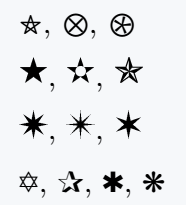# How to make a star symbol(★) in LaTeX?

Many of us use the asterisk symbol as a star symbol. However, there is a difference in shape between the two symbols. Both symbols have \ast and \star default commands.

Symbol Star
Type of symbol Star polygon
Package No
Argument No
Latex command \star
Example \star → ★

And topic of this tutorial is Star Symbol. So, this symbol’s different styles are shown below in this tutorial.

\documentclass{article}
\usepackage{MnSymbol}
\begin{document}
% Star symbol usibg MnSymbol package
$$\filledstar,\;\ostar$$
$$\thinstar,\;\smallstar$$
$$\oast$$
% Default command
$$\star$$
\end{document}

Output :## Use big star symbol in LaTeX

There is a default \bigstar command for the Big star symbol. If you want to see big star symbols of different styles then you have to take the help of an external package.

\documentclass{article}
\usepackage{MnSymbol,bbding,pifont}
\begin{document}
%star symbol using MnSymbol package
$\pentagram,\;\bigotimes,\;\bigoast$\\[6pt]
% Star symbol in text mode using bbding package
\FiveStar, \FiveStarCenterOpen, \FiveStarConvex \\[6pt]
\EightStar, \EightStarTaper, \SixStar \\[6pt]
%Star sumbol using pifont package(use \ding{65 to 107})
\ding{65}, \ding{80}, \ding{81}, \ding{107}
\end{document}

Output :You will understand when you see the code above. The number is passed as an argument in the \ding{num} command included in the pifont package.

If you want, you can pass different numbers between 65 to 107 and print star symbols of different styles in latex